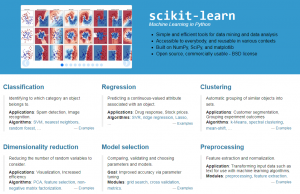# sklearn使用总结

10813℃

scikit-learn是Python实现的一个传统机器学习算法库，基本涵盖了机器学习涉及到的各个方面，包括数据的预处理、特征的提取、模型的构建、模型的训练、模型的验证以及模型的评价等等，目前项目已在Github上star数目超过2w。scikit-learn一般简称为sklearn，我对sklearn开始接触已经有4年多的时间，期间好久时间没再搞过。本文仅基于以前我对sklearn的一些使用总结展开介绍，如果与最新版本有些出入，那就以后者为准。

## sklearn的安装

``````
pip install -U scikit-learn
``````

sklearn依赖Python环境，需要预先安装NumPy和Scipy库，Matplotlib库也是必要的。

## 有监督学习与无监督学习

``````
# 分类
from sklearn.neighbors import KNeighborsClassifier
from sklearn.naive_bayes import GaussianNB, MultinomialNB
from sklearn.svm import LinearSVC, SVC
from sklearn.tree import DecisionTreeClassifier
from sklearn.ensemble import RandomForestClassifier
clf = XXXXClassifier()
clf.fit(X_train, y_train)
pred = clf.predict(X_test)
print(list(pred))
``````

``````
# 聚类
from sklearn.cluster import KMeans
clt = KMeans(n_clusters=5)
clt.fit(X_test)
print(list(clt.labels_))
``````

## 数据预处理

sklearn的数据预处理模块，可以在sklearn.preprocessing下找到，比较常用的是MinMaxScaler、StandardScaler、Normalizer等方法。

#### 特征降维与特征选取

sklearn.decomposition下提供了PCA方法，对应特征降维，sklearn.feature_selection下提供了一系列特征选取方法，比如说SelectKBest等。

## 评价指标

sklearn.metrics下提供了常见的评价指标，在评价模型的时候可以采取如下引用：

``````
from sklearn import metrics
from sklearn.metrics import \
confusion_matrix, \
classification_report, \
precision_score, \
recall_score, \
f1_score, \
accuracy_score
``````

## 关于模型参数寻优

#### 交叉验证

sklearn.cross_validation下提供了交叉验证的方法，比较常用的是train_test_split、StratifiedKFold、KFold等。

#### 网格搜索

``````
clf = GridSearchCV(
estimator = pipeline,
param_grid = parameters,
cv = StratifiedKFold(y_train, 5),
# cv = KFold(y_train, 5),
scoring = "accuracy", # or scorer
n_jobs = 3
)
clf.fit(X_train, y_train)
``````